﻿ 任意形状功能梯度板的自由振动分析 Free Vibration Analysis of Functional Gradient Mindlin Plate of Arbitrary Shape

Open Journal of Acoustics and Vibration
Vol. 07  No. 01 ( 2019 ), Article ID: 29226 , 13 pages
10.12677/OJAV.2019.71004

Free Vibration Analysis of Functional Gradient Mindlin Plate of Arbitrary Shape

Yunyan Yu1,2,3*, Xiang Zhu1,2,3, Tianyun Li1,2,3, Wenjie Guo1,4#

1School of Naval Architecture and Ocean Engineering, Huazhong University of Science and Technology, Wuhan Hubei

2Hubei Key Laboratory of Naval Architecture and Ocean Engineering Hydrodynamics, Wuhan Hubei

3Collaborative Innovation Center for Advanced Ship and Deep-Sea Exploration, Shanghai

4Engineering Research Center of Railway Environment Vibration and Noise Ministry Education, East China Jiaotong University, Nanchang Jiangxi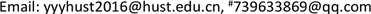Received: Feb. 16th, 2019; accepted: Mar. 6th, 2019; published: Mar. 13th, 2019ABSTRACT

In this paper, the free vibration characteristics of functionally graded plates in arbitrary shape were studied based on the improved Rayleigh Ritz method. Assuming that the material of the functionally graded plate changes exponentially along the thickness direction, the vibration of the plate is described by Mindlin plate theory and the improved Fourier series is used as the displacement tolerance function. The energy functional expressions of the functionally graded plate are derived and the natural frequency is obtained by solving them. The convergence and accuracy of the proposed method are verified by compared with the existing literature. Then the proposed method was applied to the triangular and circular plates respectively, in which the versatility of the proposed method was showing. Finally the influences of gradient index, boundary condition and thickness of the functionally graded plate on the free vibration are discussed.

Keywords:Arbitrary Shape, Functionally Graded, Typical Boundary Conditions, Rayleigh-Ritz Method, Improved Fourier Series Method

1华中科技大学船舶与海洋工程学院，湖北 武汉

2船舶与海洋水动力湖北省重点实验室，湖北 武汉

3高新船舶与深海开发装备协同创新中心，上海

4华东交通大学，铁路环境振动与噪声教育部工程研究中心，江西 南昌1. 引言

2. 理论模型

2.1. 功能梯度材料

$X\left(z\right)={X}_{m}{V}_{m}\left(z\right)+{X}_{c}{V}_{c}\left(z\right)$ (1)

${V}_{m}\left(z\right)+{V}_{c}\left(z\right)=1$ (2)

${V}_{m}={\left(\frac{2z+h}{2h}\right)}^{k}\text{\hspace{0.17em}}\text{\hspace{0.17em}}\text{\hspace{0.17em}}\left(k\ge 0\right)$ (3)

$X\left(z\right)=\left({X}_{m}-{X}_{c}\right){\left(\frac{2z+h}{2h}\right)}^{k}+{X}_{c}$ (4)

2.2. 改进的Rayleigh-Ritz法

2.2.1. 函数域的拓展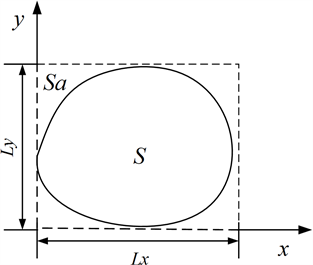Figure 1. Calculation model of arbitrary shaped plate

S为薄板的求解域，Sa为试函数的积分域，长度分别为Lx和Ly。当求解域S为任意的不规则形状时，可以假想将结构域扩展为一个略大于真实域的矩形域Sa，矩形域要涵盖待求取结构的真实求解域。一般来说，取求解域距x、y轴最大距离作为矩形域的边界。当采用改进的傅里叶级数作为结构的试函数时，使用矩形域能更便捷地构造出合理的试函数。而在求板的应变能、动能时，只需对真实求解域进行离散并积分，然后求取泛函极值得到振动方程。

2.2.2. 曲边边界的虚拟弹簧处理方法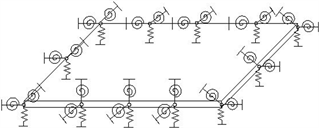Figure 2. Model of rectangular plate

3. 公式推导

$\begin{array}{l}w\left(x,y,t\right)=\sum _{m=0}^{M}\sum _{n=0}^{N}{A}_{mn}{f}_{m}\left(x\right){g}_{n}\left(y\right){\text{e}}^{i\omega t}\\ {\beta }_{x}\left(x,y,t\right)=\sum _{m=0}^{M}\sum _{n=0}^{N}{B}_{mn}{f}_{m}\left(x\right){g}_{n}\left(y\right){\text{e}}^{i\omega t}\\ {\beta }_{y}\left(x,y,t\right)=\sum _{m=0}^{M}\sum _{n=0}^{N}{C}_{mn}{f}_{m}\left(x\right){g}_{n}\left(y\right){\text{e}}^{i\omega t}\end{array}$ (4)

$\begin{array}{l}{f}_{m}\left(x\right)=\mathrm{sin}\left(\frac{m\pi }{Lx}x\right)\text{\hspace{0.17em}}\text{\hspace{0.17em}}\text{\hspace{0.17em}}\text{\hspace{0.17em}}\text{\hspace{0.17em}}\text{\hspace{0.17em}}\text{\hspace{0.17em}}\text{\hspace{0.17em}}0 (5a)

$\begin{array}{l}{g}_{n}\left(y\right)=\mathrm{sin}\left(\frac{n\text{π}}{Ly}y\right)\text{\hspace{0.17em}}\text{\hspace{0.17em}}\text{\hspace{0.17em}}\text{\hspace{0.17em}}\text{\hspace{0.17em}}\text{\hspace{0.17em}}\text{\hspace{0.17em}}\text{\hspace{0.17em}}\text{\hspace{0.17em}}0 (5b)

 (6)

$\left[\begin{array}{c}u\\ v\\ w\end{array}\right]=\left[\begin{array}{c}\left(z-{z}_{0}\right){\beta }_{x}\\ \left(z-{z}_{0}\right){\beta }_{y}\\ w\left(x,y\right)\end{array}\right]$ (7)

$\left\{\begin{array}{c}{\sigma }_{x}\\ {\sigma }_{y}\\ {\tau }_{xy}\end{array}\right\}=\frac{E\left(z\right)}{1-{\mu }^{2}}\left[\begin{array}{ccc}1& \mu & 0\\ \mu & 1& 0\\ 0& 0& \frac{1-\mu }{2}\end{array}\right]\left\{\begin{array}{c}{\epsilon }_{x}\\ {\epsilon }_{y}\\ {\gamma }_{xy}\end{array}\right\}$ (8)

$\left\{\begin{array}{c}{\tau }_{yz}\\ {\tau }_{xz}\end{array}\right\}=G\left[\begin{array}{cc}1& 0\\ 0& 1\end{array}\right]\left\{\begin{array}{c}{\gamma }_{yz}\\ {\gamma }_{xz}\end{array}\right\}$ (9)

$\begin{array}{l}{M}_{x}=-{\int }_{-\frac{h}{2}}^{\frac{h}{2}}{\sigma }_{x}\left(z-{z}_{0}\right)\text{d}z\\ {M}_{y}=-{\int }_{-\frac{h}{2}}^{\frac{h}{2}}{\sigma }_{y}\left(z-{z}_{0}\right)\text{d}z\\ {M}_{xy}=-{\int }_{-\frac{h}{2}}^{\frac{h}{2}}{\tau }_{xy}\left(z-{z}_{0}\right)\text{d}z\\ {Q}_{x}={\int }_{-\frac{h}{2}}^{\frac{h}{2}}{\tau }_{xz}\left(z-{z}_{0}\right)\text{d}z\\ {Q}_{y}={\int }_{-\frac{h}{2}}^{\frac{h}{2}}{\tau }_{yz}\left(z-{z}_{0}\right)\text{d}z\end{array}\right\}$ (10)

$\left\{\begin{array}{c}{M}_{x}\\ {M}_{y}\\ {M}_{xy}\\ {Q}_{x}\\ {Q}_{y}\end{array}\right\}=\left[\begin{array}{cc}{G}_{b}& \\ & {G}_{s}\end{array}\right]\left\{\begin{array}{c}\frac{\partial {\beta }_{x}}{\partial x}\\ \frac{\partial {\beta }_{y}}{\partial y}\\ \frac{\partial {\beta }_{x}}{\partial y}+\frac{\partial {\beta }_{y}}{\partial x}\\ \frac{\partial w}{\partial x}-{\beta }_{x}\\ \frac{\partial w}{\partial y}-{\beta }_{y}\end{array}\right\}$ (11)

${G}_{b}=-{\int }_{-\frac{h}{2}}^{\frac{h}{2}}\frac{E\left(z\right){\left(z-{z}_{0}\right)}^{2}}{1-{\mu }^{2}}\left[\begin{array}{ccc}1& \mu & 0\\ \mu & 1& 0\\ 0& 0& \frac{1-\mu }{2}\end{array}\right]\text{d}z$ (12)

${G}_{s}={k}_{0}{\int }_{-\frac{h}{2}}^{\frac{h}{2}}\left[\begin{array}{cc}G& 0\\ 0& G\end{array}\right]\text{d}z$ (13)

${d}^{\text{T}}=\left[\begin{array}{ccc}{A}_{mn}& {B}_{mn}& {C}_{mn}\end{array}\right]$ (14)

${\lambda }^{\text{T}}=\left[{f}_{1}\left(x\right){g}_{1}\left(y\right),{f}_{1}\left(x\right){g}_{2}\left(y\right),\cdots ,{f}_{1}\left(x\right){g}_{N}\left(y\right),\cdots ,{f}_{M}\left(x\right){g}_{N}\left(y\right)\right]$ (15)

$\left[\begin{array}{l}w\\ u\\ v\end{array}\right]=\left[\begin{array}{ccc}{\lambda }^{\text{T}}& 0& 0\\ 0& \left(z-{z}_{0}\right){\lambda }^{\text{T}}& 0\\ 0& 0& \left(z-{z}_{0}\right){\lambda }^{\text{T}}\end{array}\right]d=Pd$ (16)

$T={\iint }_{S}{P}^{\text{T}}{m}_{0}P\text{d}x\text{d}y$ (17)

${m}_{0}={\int }_{-\frac{h}{2}}^{\frac{h}{2}}\rho \left(z\right)\left[\begin{array}{ccc}z& 0& 0\\ 0& {z}^{2}& 0\\ 0& 0& {z}^{2}\end{array}\right]\text{d}z$ (18)

${V}_{p}=\frac{1}{2}{\iint }_{s}{B}^{\text{T}}\left[\begin{array}{cc}{G}_{b}& 0\\ 0& {G}_{s}\end{array}\right]B\text{d}x\text{d}y$ (19)

$B=\left[\begin{array}{ccc}0& \frac{\partial \lambda }{\partial x}& 0\\ 0& 0& \frac{\partial \lambda }{\partial y}\\ 0& \frac{\partial \lambda }{\partial y}& \frac{\partial \lambda }{\partial x}\\ \frac{\partial \lambda }{\partial x}& \lambda & 0\\ \frac{\partial \lambda }{\partial y}& 0& \lambda \end{array}\right]$ (20)

${K}_{s}=\left[\begin{array}{ccc}{k}_{l}& 0& 0\\ 0& {K}_{xl}& 0\\ 0& 0& {K}_{yl}\end{array}\right]$ (21)

${V}_{s}=\frac{1}{2}{\int }_{l}{\lambda }^{\text{T}}{K}_{s}\lambda \text{d}l$ (22)

$\prod ={V}_{p}+{V}_{s}-T$ (23)

$\begin{array}{l}\frac{\partial \prod }{\partial {B}_{mn}}=0\\ \frac{\partial \prod }{\partial {A}_{mn}}=0\\ \frac{\partial \prod }{\partial {C}_{mn}}=0\end{array}\right\}$ (24)

$\left(K-{\omega }^{2}M\right)A=0$ (25)

4. 数值计算

4.1. 材料属性

4.2. 收敛性分析Table 3. Natural frequencies of rectangular plate (Hz), F-F-F-F

Table 4. Natural frequencies of rectangular plate (Hz), C-C-C-C

4.3. 准确性验证Table 5. Natural frequency parameters of rectangular plate, (S-S-S-S, Al/Al2O3, a/b = 1)

4.4. 功能梯度三角形板的弯曲自由振动特性分析Table 7. First six natural frequency parameters of triangular plate under different gradient index

4.5. 功能梯度圆形板的弯曲自由振动分析Table 8. First six natural frequency parameters of circle plate under different gradient index

4.6. 梯度指数k对功能梯度板的固有频率影响分析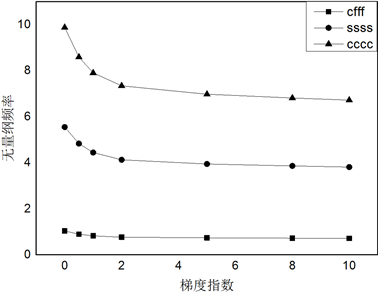Figure 3. Curves of the first-order dimensionless natural frequency of rectangular FGM plate with gradient index under different boundary conditions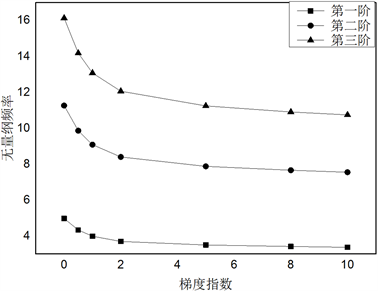Figure 4. Curves of the first three-order dimensionless natural frequency of rectangular FGM plate, S-S-S-S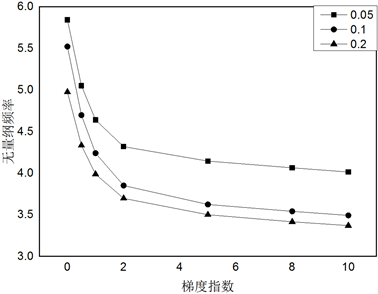Figure 5. Curves of the first-order dimensionless natural frequency of rectangular FGM plate with gradient index under different thicknesses

5. 结论

Free Vibration Analysis of Functional Gradient Mindlin Plate of Arbitrary Shape[J]. 声学与振动, 2019, 07(01): 28-40. https://doi.org/10.12677/OJAV.2019.71004

1. 1. Niino, M. (1987) Functionally Gradient Materials as Thermal Barrier for Space Plane. Journal of the Japan Society for Composite Ma-terials, 13, 257-264. ://doi.org/10.6089/jscm.13.257

2. 2. Koizumi, M. (1993) The Concept of FGM. Cerzmic Trans. Function-ally Gradient Materials, 34, 3-10.

3. 3. 李华东, 朱锡, 梅志远, 张颖军. 功能梯度板壳的力学研究进展[J]. 材料导报, 2012, 26(1): 110-118.

4. 4. 王明禄, 魏高峰, 李翠艳. 功能梯度材料梁的自由振动问题研究[J]. 山东轻工业学院学报(自然科学版), 2009, 23(3): 19-21.

5. 5. 曹志远. 功能梯度复合材料圆柱壳基本理论及长壳固有振动解[J]. 玻璃钢/复合材料, 2006(4): 3-6.

6. 6. Khov, H., Li, W.L. and Gibson, R.F. (2009) An Accurate Solution Method for the Static and Dynamic Deflections of Ortho-tropic Plates with General Boundary Conditions. Composite Structures, 90, 474-481. ://doi.org/10.1016/j.compstruct.2009.04.020

7. 7. 徐坤, 陈美霞, 谢坤. 正交各向异性功能梯度材料平板振动分析[J]. 噪声与振动控制, 2016, 36(4): 14-20.

8. 8. 梁斌, 李戎, 张伟, 徐红玉. 功能梯度材料圆柱壳的振动特性研究[J]. 船舶力学, 2011, 15(Z1): 109-117.

9. 9. 李伟柏, 曹志远, 唐寿高. 正交各向异性功能梯度材料开口圆柱壳的自由振动分析[J]. 力学季刊, 2016, 37(3): 433-440.

10. 10. 陈淑萍, 赵红晓, 耿少波, 武晋文. 功能梯度材料Timoshenko型剪切梁的自由振动分析[J]. 材料科学与工程学报, 2018, 36(1): 112-116.

11. 11. 尹硕辉, 余天堂, 刘鹏. 基于等几何有限元法的功能梯度板自由振动分析[J]. 振动与冲击, 2013, 32(24): 180-186.

12. 12. Bhangale, R.K. and Ganesan, N. (2006) Free Vibration of Simply Supported Functionally Graded and Layered Magneto-Electro-Elastic Plates by Finite Element Method. Journal of Sound & Vibration, 294, 1016-1038. ://doi.org/10.1016/j.jsv.2005.12.030

13. 13. 王青山, 史冬岩, 罗祥程. 任意边界条件下矩形板的面内自由振动特性[J]. 华南理工大学学报(自然科学版), 2015, 43(6): 127-134.

14. 14. Gilhooley, D.F., Batra, R.C., Xiao, J.R., et al. (2007) Analysis of Thick Functionally Graded Plates by Using Higher-Order Shear and Normal Deformable Plate Theory and MLPG Method with Radial Basis Functions. Composite Structures, 80, 539-552. ://doi.org/10.1016/j.compstruct.2006.07.007

15. 15. Zhao, X., Lee, Y.Y. and Liew, K.M. (2009) Free Vibration Analysis of Functionally Graded Plates Using the Element-Free kp-Ritz Method. Journal of Sound and Vibration, 319, 918-939. ://doi.org/10.1016/j.jsv.2008.06.025

16. 16. Hosseini-Hashemi, Sh., Rokni Damavandi Taher, H., Akha-van, H. and Omidi, M. (2010) Free Vibration of Functionally Graded Rectangular Plates Using First-Order Shear Deformation Plate Theory. Applied Mathematical Modelling, 34, 1276-1291. ://doi.org/10.1016/j.apm.2009.08.008